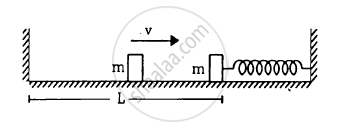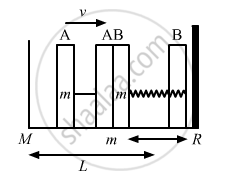Advertisement Remove all ads

# The Left Block in Figure Moves at a Speed V Towards the Right Block Placed in Equilibrium. All Collisions to Take Place Are Elastic and the Surfaces Are Frictionless. - Physics

Sum

The left block in figure moves at a speed v towards the right block placed in equilibrium. All collisions to take place are elastic and the surfaces are frictionless. Show that the motions of the two blocks are periodic. Find the time period of these periodic motions. Neglect the widths of the blocks.Advertisement Remove all ads

#### SolutionAccording to the question, the collision is elastic and the surface is frictionless, therefore, when the left block A moves with speed v and collides with the right block B, it transfers all the energy to the right block B.
The left block A moves a distance x against the spring; the right block returns to the original position and completes half of the oscillation.

Therefore, the period of right block B will be, $T = \frac{2\pi\sqrt{\left( \frac{m}{k} \right)}}{2} = \pi\sqrt{\left( \frac{m}{k} \right)}$

Right block B collides with left block A and comes to rest.
Let L be the distance moved by the block to return to its original position.
The time taken is given by,

$\frac{L}{V} + \frac{L}{V} = 2\left( \frac{L}{V} \right)$

Hence, time period of the periodic motion is,

$2\frac{L}{V} + \pi\sqrt{\left( \frac{m}{k} \right)}$
Is there an error in this question or solution?
Advertisement Remove all ads

#### APPEARS IN

HC Verma Class 11, 12 Concepts of Physics 1
Chapter 12 Simple Harmonics Motion
Q 28 | Page 254
Advertisement Remove all ads

#### Video TutorialsVIEW ALL 

Advertisement Remove all ads
Share
Notifications

View all notifications

Forgot password?